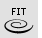# FitCrv

Toolbar MenuCurve

Curve Edit Tools >

Refit to Tolerance

The FitCrv command makes a non-rational NURBS curve of a specified degree that matches the input curve to within the specified tolerance.

#### Note

• Generally, use this command to fit very dense or complex curves to a simpler structure.
• The parameterization is chosen so that the domain is approximately from 0 to the curve's length and for a parameter t in the domain, the length of the sub-curve from 0 to t is approximately equal to t.
• Knot spacing is based on the curvature of the input curve, so knots are closer together in areas of higher curvature.
• The resulting curve may have fewer control points than the input, or it may have more points if the input is simple.

#### Steps

1. Select curves.
2. Type a new tolerance value or press Enter.
You can also pick two points to set the tolerance.
3. Type 0 (zero) to use the current absolute tolerance.

#### Note

• When the input to the FitCrv command is a polyline, the FitCrv command treats the polyline vertices as a list of points, and it tries to compute a curve that goes near the points but has a reasonable number of control points. The FitCrv command is meant for polylines with many closely spaced points.
• When the input to the FitCrv command is a control points wiggly curve with many control points, the FitCrv command tries to compute a curve that has the same general shape but fewer control points.
Command-line options

DeleteInput

##### Yes

Deletes the original geometry.

##### No

Retains the original geometry.

Degree

Specifies the degree of the curve (or surface).

When drawing a high-degree curve, the output curve will not be the degree you request unless there is at least one more control point than the degree.

OutputLayer

Specifies the layer for the results of the command.

##### Current

Places the results on the current layer.

##### Input

Places the results on the same layer as the input curve.

Note: If the input object is in a model that is attached using Worksession, the object will be placed on the current layer.

##### TargetObject

Places the results on the same layer as the target surface.

AngleTolerance

When a kink in the input curve is this angle or less from tangent, the kink will be refit smoothly, otherwise the output curve will also have a kink at that location.

## See also

Rhino for Mac © 2010-2017 Robert McNeel & Associates. 24-Oct-2017